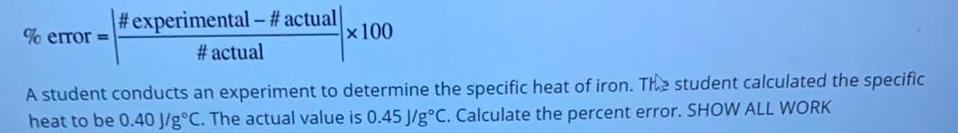Question:

# A student conducts an experiment to determine the specific

Last updated: 7/24/2022A student conducts an experiment to determine the specific heat of iron. The student calculated the specific heat to be 0.40 J/g°C. The actual value is 0.45 J/g°C. Calculate the percent error. SHOW ALL WORK